Publicaciones

## document.write(ultimaFecha);

Dentro de los parámetros fundamentales que debemos considerar al momento de ensayar o seleccionar un capacitor electrolítico es el valor de su Resistencia Serie Equivalente (ESR). En el mundo de las reparaciones, una enorme cantidad de fallas presentes en los equipos electrónicos son generadas por los capacitores electrolíticos y la pérdida de su apropiada ESR. Nos podemos encontrar con capacitores que poseen un valor muy bueno (o aproximado al nominal) de su capacidad  y sin embargo son los causantes de miles de problemas e inconvenientes por haber perdido su ESR.

La teoría nos indica que un capacitor real difiere bastante de un modelo ideal donde sólo existe un valor de capacidad. Como te mostramos en la imagen anterior, un capacitor real está compuesto por inductancias y resistencias propias de los materiales y el tipo de construcción que poseen estos dispositivos. En un aspecto elemental, la práctica nunca se contradice con la teoría y es en la existencia de un dieléctrico que separa las “placas” que forman el capacitor. El calor es uno de los factores fundamentales que deterioran el dieléctrico de un capacitor. Además, por pequeños que sean los valores de EPR y ESR son valores que nunca son iguales a cero. Por este motivo siempre, al circular corriente por un capacitor, existirá una disipación de potencia en forma de calor. Cuanto mayor sea el rizado al que esté expuesto un capacitor y mayores sean las resistencias serie y paralelo, mayor será la temperatura que alcance. Este fenómeno provocará una degradación paulatina del dieléctrico que llevará a una elevación gradual de las componentes resistivas. Esta espiral destructiva provoca con el tiempo fallas, a veces insólitas, que no siempre son fáciles de resolver. El medidor de ESR (ESR Meter) es el instrumento que ayuda a resolver estos problemas de manera muy sencilla y eficaz.

Más Sobre ESR.

The basics of capacitors are explained in this technical column.
Today's column describes frequency characteristics of the amount of impedance |Z| and equivalent series resistance (ESR) in capacitors.
Understanding frequency characteristics of capacitors enables you to determine, for example, the noise suppression capabilities or the voltage fluctuation control capabilities of a power supply line. Frequency characteristics are therefore important parameters that are essential for circuit design. This column describes two types of frequency characteristics: impedance |Z| and ESR.

1.Frequency characteristics of capacitors
The impedance Z of an ideal capacitor (Fig. 1) is shown by formula (1), where ω is the angular frequency and C is the electrostatic capacitance of the capacitor.Figure 1. Ideal capacitorFrom formula (1), the amount of impedance |Z| decreases inversely with the frequency, as shown in Figure 2. In an ideal capacitor, there is no loss and the equivalent series resistance (ESR) is zero.Figure 2. Frequency characteristics of an ideal capacitor

In actual capacitors (Fig. 3), however, there is some resistance (ESR) from loss due to dielectric substances, electrodes or other components in addition to the capacity component C and some parasitic inductance (ESL) due to electrodes, leads and other components. As a result, the frequency characteristics of |Z| form a V-shaped curve (or U-shaped curve depending on the type of capacitor) as shown in Figure 4, and ESR also shows frequency characteristics for values equivalent to loss.Figure 3. An actual capacitorFigure 4. An example of |Z|/ESR frequency characteristics of an actual capacitor

The reason why |Z| and ESR form curves like those shown in Figure 4 can be explained as follows.

Low-frequency region: |Z| in regions with a low frequency decreases inversely with frequency, similar to the ideal capacitor. ESR shows a value equivalent to dielectric loss from delay of polarization in the dielectric substance.
Near the resonance point: As the frequency rises, ESR resulting from parasitic inductance, electrode resistivity and other factors causes |Z| behavior to stray from that of an ideal capacitor (red broken line) and reach a minimum value. The frequency at which |Z| is the minimum value is called the self-resonant frequency, and at this time, |Z|=ESR. Once the self-resonant frequency is exceeded, the element characteristic changes from capacitor to inductor, and |Z| starts to increase. The region below the self-resonant frequency is called the capacitive region and the region above is called the inductive region.
ESR is affected by loss caused by the electrode in addition to dielectric loss.
High-frequency region: In frequency zones even higher than the resonance point, |Z| characteristics are determined by parasitic inductance (L). |Z| in the high-frequency region approaches formula (2) and increases proportionately with frequency.
As for ESR, electrode skin effects, proximity effects and other effects begin to appear.The above was an explanation of frequency characteristics of an actual capacitor. The main point to remember is that, as frequency rises, ESR and ESL cannot be ignored. As there is an increasing number of applications in which capacitors are used at high frequencies, ESR and ESL become an important parameter that shows capacitor performance, in addition to electrostatic capacitance values.

# Dissipation factor

In physics, the dissipation factor (DF) is a measure of loss-rate of energy of a mode of oscillation (mechanical, electrical, or electromechanical) in a dissipative system. It is the reciprocal of quality factor, which represents the "quality" or durability of oscillation.

## Explanation

Electrical potential energy is dissipated in all dielectric materials, usually in the form of heat. In a capacitor made of a dielectric placed between conductors, the typical lumped element model includes a lossless ideal capacitor in series with a resistor termed the equivalent series resistance (ESR) as shown below. The ESR represents losses in the capacitor. In a good capacitor the ESR is very small, and in a poor capacitor the ESR is large. However, ESR is sometimes a minimum value to be required. Note that the ESR is not simply the resistance that would be measured across a capacitor by an ohmmeter. The ESR is a derived quantity with physical origins in both the dielectric's conduction electrons and dipole relaxation phenomena. In a dielectric only one of either the conduction electrons or the dipole relaxation typically dominates loss. For the case of the conduction electrons being the dominant loss, then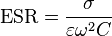$\text{ESR} = \frac {\sigma} {\varepsilon \omega^2 C}$
where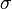$\sigma$ is the dielectric's bulk conductivity,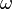$\omega$ is the angular frequency of the AC current i,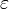$\varepsilon$ is the lossless permittivity of the dielectric, and$C$ is the lossless capacitance.A real capacitor has a lumped element model of a lossless ideal capacitor in series with an equivalent series resistance (ESR). The loss tangent is defined by the angle between the capacitor's impedance vector and the negative reactive axis.
If the capacitor is used in an AC circuit, the dissipation factor due to the non-ideal capacitor is expressed as the ratio of the resistive power loss in the ESR to the reactive power oscillating in the capacitor, or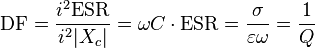$\text{DF} = \frac {i^2 \text{ESR}} {i^2 |X_{c}|} = \omega C \cdot \text{ESR} = \frac {\sigma} {\varepsilon \omega} = \frac{1}{Q}$
When representing the electrical circuit parameters as vectors in a complex plane, known as phasors, a capacitor's dissipation factor is equal to the tangent of the angle between the capacitor's impedance vector and the negative reactive axis, as shown in the diagram to the right. This gives rise to the parameter known as the loss tangent δ where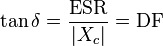$\tan\delta = \frac{\text{ESR}}{\left|X_c\right|} = \text{DF}$
Since the DF in a good capacitor is usually small, δ ~ DF, and DF is often expressed as a percentage.
DF approximates to the power factor when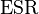$\text{ESR}$ is far less than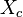$X_c$, which is usually the case.
DF will vary depending on the dielectric material and the frequency of the electrical signals. In low dielectric constant (low-k), temperature compensating ceramics, DF of 0.1% to 0.2% is typical. In high dielectric constant ceramics, DF can be 1% to 2%. However, lower DF